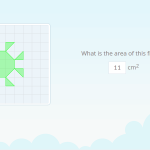Smartick is a fun way to learn math!# I want to learn about: Geometry

Learn elementary geometry with Smartick. Exercises, theory, tutorials, and teaching resources for children learning elementary geometry. Here you will find posts about geometry to make learning easier and more fun.

Jun27

## What is a Prism? Solve these Exercises to Find OutWelcome to this post about prisms! Have you ever wondered what is a prism? Look no further! In this guide, we will explain everything you need to know about prisms. Is this figure a prism? Before we dive into the fascinating world of these three-dimensional geometric figures, we are going to test your skills with […]

Mar03

## How to Calculate the Area?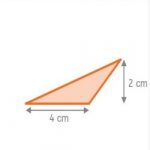Geometry is seen in school as a collection of formulas that answer the question, how to calculate the area? Geometry is much more than that: it comprises the whole part of mathematics that solves problems of space, answers questions about the shape of plane and three-dimensional figures and, it goes much further, trying to answer […]

Feb09

## Geometric Shapes with ExamplesToday you will learn about geometric shapes. We are going to teach you about the characteristics of the main geometric shapes, and to help you understand better, we will explain with examples. What Are Geometric Shapes? The ancient Greeks defined straight and curved lines by looking at their environment. Do you think they stopped there? […]

Mar11

## Consecutive Interior Angles: What They Are and How to Find Them With Examples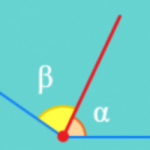In this post, we are going to discover what consecutive interior angles are and how to differentiate them from other types of angles. You will see some examples and even a little quiz. But before we start, let’s review what an angle is. An angle is the portion of a plane between two rays that […]

Jan15

## What is an Obtuse Angle?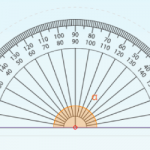In today’s post, we are going to learn about what an obtuse angle is with different examples taken from everyday life and some Smartick exercises. What is an Obtuse Angle? An angle is a space shared by two rays that are joined at the same vertex. We can find different types of angles according to […]

Dec28

## Complementary Angles: What Are They?In today’s post, we are going to learn about what complementary angles are and their properties. After, we will look at some examples and complete a few exercises together. Before we begin, we would recommend reviewing the types of angles that exist. What Are Complementary Angles? Two angles are complementary if the sum of the […]

Nov26

## Perimeter: What Is It and How to Find It for Any Polygon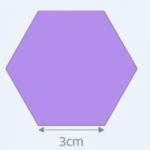Today we are going to learn to calculate perimeters of geometric figures. But first, let’s start with the definition. What is a Perimeter? A perimeter is what we call the length of the outline of a geometric figure. Therefore, the perimeter is a measurement of length, so it will come in centimeters, inches, and feet, […]

Oct30

## What is a Straight Angle and ExamplesIn today’s post, we will learn what a straight angle is, how many degrees it has, and its properties. We will also practice with some examples. What is a Straight Angle? An angle is a portion of the plane between two rays that are joined by a vertex. A straight angle is the shared space […]

Apr13

## Elementary Geometry – Open LinesIn this post, we are going to discover what an open line is and how it is different from a closed line. We will also see different types of open lines. Before we begin, take a look at this post about straight and curved lines to refresh your memory. Open Lines We know that a […]

Mar23

## Identifying Flat Symmetrical Figures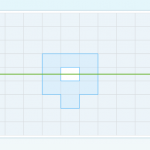In today’s post, I am going to present a series of exercises to practice how to identify flat symmetrical figures. To begin, I recommend that if you don’t remember symmetry or the characteristics that make a figure symmetrical very well, take a look at this previous post. You’ll be able to review all of the […]

Jan06

## How to Calculate a Perimeter: Part 2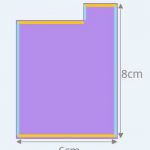In an earlier post, we learned how to calculate the perimeter of any polygon and formulas for regular polygons and rectangles. Today, we are going to learn how to calculate the perimeter of other types of polygons: rhombuses, isosceles triangles, isosceles trapezoids, and stepped polygons. NOTE TO OUR READERS IN THE U.K. – This post […]